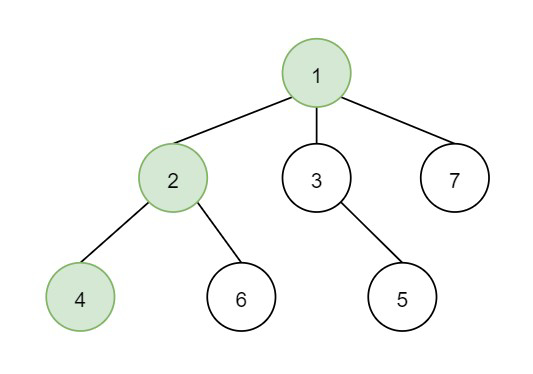# Frog Position After T Seconds in C++

Suppose we have one undirected tree consisting of n vertices. The vertices are numbered from 1 to n. Now a frog starts jumping from the vertex 1. The frog can jump from its current vertex to another non-visited vertex if they are adjacent, in one second. The frog can not jump back to a visited vertex. In case the frog can jump to several vertices it jumps randomly to one of them

where the probability is same, otherwise, when the frog can not jump to any non-visited vertex it jumps forever on the same vertex.

The tree is given as an array of edges. We have to find the probability that after t seconds the frog is on the vertex target.

So, if the input is like n is 7, t is 2, target is 4 and the tree is like −then the output will be 0.1666, as from the graph. The frog starts at vertex 1, jumping with 0.3333 probability to the vertex 2 after second 1 and then jumping with 0.5 probability to vertex 4 after second 2. Thus the probability for the frog is on the vertex 4 after 2 seconds is 0.3333 * 0.5 = 1.6665.

To solve this, we will follow these steps −

• ret := 1

• Define one set visited

• Define a function dfs(), this will take node, start, a list of edges g, time, t, one stack st,

• if node is member of visited, then −

• return false

• insert node into visited

• if node is same as 1, then −

• tt := time, ok := true

• return true

• for initialize i := 0, when i < size of g[node], update (increase i by 1), do −

• insert g[node, i] into st

• if dfs(g[node, i], start, g, time + 1, t, st) is true, then −

• return true

• delete element from st

• return false

• From the main method do the following −

• ret := 1

• ok := false

• Define an array of lists graph of size n + 1

• Define an array of lists graph2 of size n + 1

• for initialize i := 0, when i < size of edges, update (increase i by 1), do −

• insert edges[i, 1] at the end of graph[edges[i, 0]]

• insert edges[i, 0] at the end of graph[edges[i, 1]]

• Define one stack st

• dfs(target, target, graph, 0, t, st)

• while (not st is empty), do −

• node := top element of st

• sz := size of graph[node]

• if node is not equal to 1, then −

• (decrease sz by 1)

• ret := ret * (1.0 / sz)

• delete element from st

• if tt > t, then −

• return 0

• if tt is same as t, then −

• return ret

• if tt < t and target is same as 1 and size of graph[target] >= 1, then −

• return 0

• return (if tt < t and size of graph[target] > 1, then 0, otherwise ret)

Let us see the following implementation to get better understanding −

## Example

Live Demo

#include <bits/stdc++.h>
using namespace std;
class Solution {
public:
double ret = 1;
bool ok;
set<int> visited;
int tt;
bool dfs(int node, int start, vector<int> g[], int time, int t,
stack<int>& st){
if (visited.count(node))
return false;
visited.insert(node);
if (node == 1) {
tt = time;
ok = true;
return true;
}
for (int i = 0; i < g[node].size(); i++) {
st.push(g[node][i]);
if (dfs(g[node][i], start, g, time + 1, t, st))
return true;
;
st.pop();
}
return false;
}
double frogPosition(int n, vector<vector<int> >& edges, int t,
int target){
ret = 1;
ok = false;
vector<int> graph[n + 1];
vector<int> graph2[n + 1];
for (int i = 0; i < edges.size(); i++) {
graph[edges[i]].push_back(edges[i]);
graph[edges[i]].push_back(edges[i]);
}
stack<int> st;
dfs(target, target, graph, 0, t, st);
while (!st.empty()) {
int node = st.top();
double sz = (double)graph[node].size();
if (node != 1)
sz--;
ret *= (1.0 / sz);
st.pop();
}
if (tt > t)
return 0;
if (tt == t)
return ret;
if (tt < t && target == 1 && graph[target].size() >= 1)
return 0;
return tt < t && graph[target].size() > 1 ? 0 : ret;
}
};
main(){
Solution ob;
vector<vector<int>> v = {{1,2},{1,3},{1,7},{2,4},{2,6},{3,5}};
cout << (ob.frogPosition(7,v,2,4));
}

## Input

7, {{1,2},{1,3},{1,7},{2,4},{2,6},{3,5}}, 2, 4

## Output

0.166667